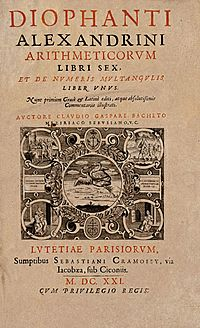# Diophantus facts for kids

Kids Encyclopedia FactsTitle page of the 1621 edition of Diophantus' Arithmetica, translated into Latin by Claude Gaspard Bachet de Méziriac.

Diophantus of Alexandria (Ancient Greek: Διόφαντος ὁ Ἀλεξανδρεύς; born probably sometime between AD 201 and 215; died around 84 years old, probably sometime between AD 285 and 299) was an Alexandrian Hellenistic mathematician, who was the author of a series of books called Arithmetica, many of which are now lost. Sometimes called "the father of algebra", his texts deal with solving algebraic equations.

While reading Claude Gaspard Bachet de Méziriac's edition of Diophantus' Arithmetica, Pierre de Fermat concluded that a certain equation considered by Diophantus had no solutions, and noted in the margin without elaboration that he had found "a truly marvelous proof of this proposition," now referred to as Fermat's Last Theorem. This led to tremendous advances in number theory, and the study of Diophantine equations ("Diophantine geometry") and of Diophantine approximations remain important areas of mathematical research. Diophantus coined the term παρισότης (parisotes) to refer to an approximate equality. This term was rendered as adaequalitas in Latin, and became the technique of adequality developed by Pierre de Fermat to find maxima for functions and tangent lines to curves. Diophantus was the first Greek mathematician who recognized fractions as numbers; thus he allowed positive rational numbers for the coefficients and solutions. In modern use, Diophantine equations are usually algebraic equations with integer coefficients, for which integer solutions are sought.Diophantus Facts for Kids. Kiddle Encyclopedia.